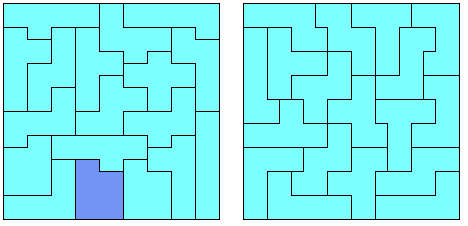## One-sided Truncated Pentominoes

Here are the 35 pieces which were suggested by Andrew Clarke. I rotated the pieces so that the long sides of the truncated squares are horizontal. They are divided up into four sets according the the maximum length which can be contributed to a straight horizontal border if rotated as shown.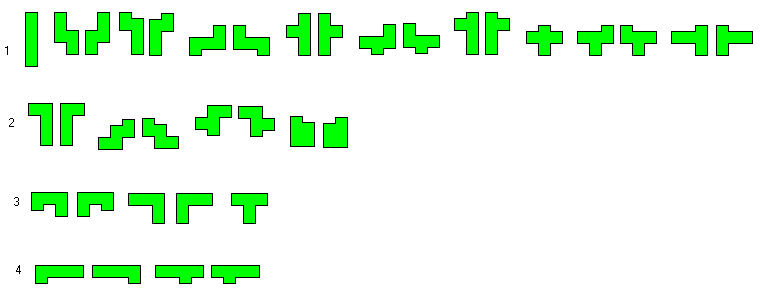The total area is 35x4.5 = 630 * 1/2 * 1/2 which can be factorised as 630 = 21x30 = 18x35 = 15x42 = 14x45 = 10x63 = 9x70 = 7x90 = 6x105 = ...

So we can ask for a lot of rectangles: 10.5x15; 9x17.5; 7.5x21; 7x22.5; 5x31.5; 4.5x35; 3.5x45; 3x52.5 ...

The first five cases are shown and the other ones are impossible because the long border can't be be covered by the pieces. Let's have look at the 4.5x35 rectangle. All 35 pieces must be rotated so that the truncated squares are "parallel" to the long border because in each column a 0.5 contribution must be found. With this orientation we have only a border contrubution of 18*1+1+8*2+5*3+4*4=66 which isn't enough for the 70 border squares at the long sides.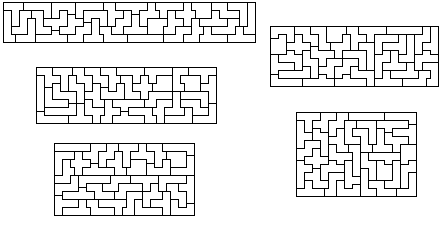Patrick Hamlyn managed to find three rectangles with the set: two 9x6 and one 9x5.5 . Beside the mirror construction there is only one other dissection of the whole set to make these three rectangles.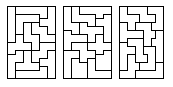Since 35 = 6^2 - 1 we can try to make 6-fold replicas of the pieces with a hole shaped like the replicated piece. There are 16 + 3 problems because we have three symmetric pieces and 16 mirror pairs.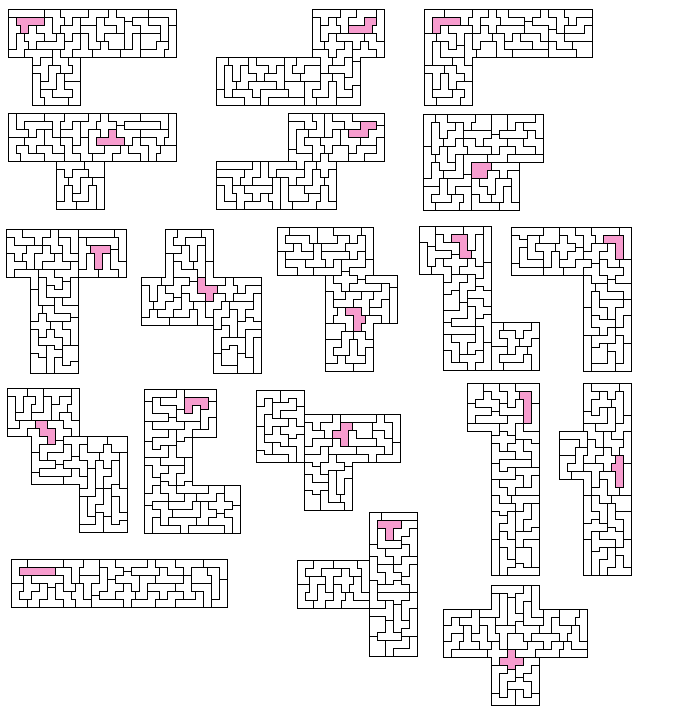If we are allowed to use one piece twice we can look for two 9x9 squares. One construction with a duplicated P-piece is shown but the duplication of other pieces might be possible as well. For hand solvers it would be a good idea to add 9 truncated squares as single pieces to simplify the construction.